GeeksforGeeks App
Open AppBrowser
Continue

# C Program to read contents of Whole File

C programming language supports four pre-defined functions to read contents from a file, defined in stdio.h header file:

1. fgetc() This function is used to read a single character from the file.
2. fgets() This function is used to read strings from files.
3. fscanf() This function is used to read formatted input from a file.
4. fread() This function is used to read the block of raw bytes from files. This is used to read binary files.

• Open a file using the function fopen() and store the reference of the file in a FILE pointer.
• Read contents of the file using any of these functions fgetc(), fgets(), fscanf(), or fread().
• File close the file using the function fclose().

Let’s begin discussing each of these functions in detail.

fgetc()

fgetc() reads characters pointed by the function pointer at that time. On each successful read, it returns the character (ASCII value) read from the stream and advances the read position to the next character. This function returns a constant EOF (-1) when there is no content to read or an unsuccessful read.

Syntax:

int fgetc(FILE *ptr);

Approach:

• This program reads the whole content of the file, using this function by reading characters one by one.
• Do-While loop will be used which will read character until it reaches to the end of the file.
• When it reaches end it returns  EOF character (-1).

Using EOF:
Below is the C program to implement the above approach-

## C

 `// C program to implement``// the above approach``#include ``#include ``#include ` `// Driver code``int` `main()``{``    ``FILE``* ptr;``    ``char` `ch;` `    ``// Opening file in reading mode``    ``ptr = ``fopen``(``"test.txt"``, ``"r"``);` `    ``if` `(NULL == ptr) {``        ``printf``(``"file can't be opened \n"``);``    ``}` `    ``printf``(``"content of this file are \n"``);` `    ``// Printing what is written in file``    ``// character by character using loop.``    ``do` `{``        ``ch = ``fgetc``(ptr);``        ``printf``(``"%c"``, ch);` `        ``// Checking if character is not EOF.``        ``// If it is EOF stop reading.``    ``} ``while` `(ch != EOF);` `    ``// Closing the file``    ``fclose``(ptr);``    ``return` `0;``}`

Input File:

`GeeksforGeeks | A computer science portal for geeks`

Output: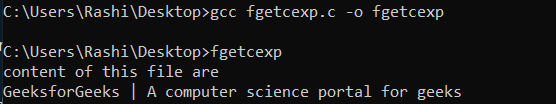In the above code, the approach is to read one character from the file and check if it is not EOF, if it is not then print it and if it is then stop reading.

Using feof():
feof() function takes file pointer as argument and returns true if pointer reaches the end of the file.

Syntax:

int feof(FILE *ptr);

Approach:

• In this approach, a character is read using fgetc().
• Using feof() function check for end of file. since feof() returns true after it reaches the end.
• Use logical NOT operator(!) so that when it reaches end condition become false and loop stop.

Below is the C program to implement the above approach:

## C

 `// C program to implement``// the above approach``#include ``#include ``#include ` `// Driver code``int` `main()``{``    ``FILE``* ptr;``    ``char` `ch;``    ``ptr = ``fopen``(``"test.txt"``, ``"r"``);` `    ``if` `(NULL == ptr) {``        ``printf``(``"file can't be opened \n"``);``    ``}` `    ``printf``(``"content of this file are \n"``);` `    ``while` `(!``feof``(ptr)) {``        ``ch = ``fgetc``(ptr);``        ``printf``(``"%c"``, ch);``    ``}``    ``fclose``(ptr);``    ``return` `0;``}`

Input File:

`GeeksforGeeks | A computer science portal for geeks`

Output: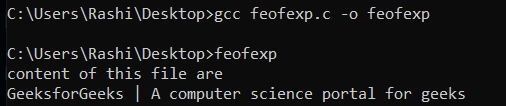fgets()

fgets() reads one string at a time from the file. fgets() returns a string if it is successfully read by function or returns NULL if can not read.

Syntax:

char * fgets(char *str, int size, FILE * ptr);

Here,
str: It is string in which fgets() store string after reading it from file.
size: It is maximum characters to read from stream.
ptr: It is file pointer.

Approach:

• In this approach, the contents of the file are read one character at a time until we reach the end of the file.
• When we reach the end of the file fgets() can’t read and returns NULL and the program will stop reading.

Below is the C program to implement the above approach:

## C

 `// C program to implement``// the above approach``#include ``#include ``#include ` `// Driver code``int` `main()``{``    ``FILE``* ptr;``    ``char` `str;``    ``ptr = ``fopen``(``"test.txt"``, ``"a+"``);` `    ``if` `(NULL == ptr) {``        ``printf``(``"file can't be opened \n"``);``    ``}` `    ``printf``(``"content of this file are \n"``);` `    ``while` `(``fgets``(str, 50, ptr) != NULL) {``        ``printf``(``"%s"``, str);``    ``}` `    ``fclose``(ptr);``    ``return` `0;``}`

Input File:

`GeeksforGeeks | A computer science portal for geeks`

Output: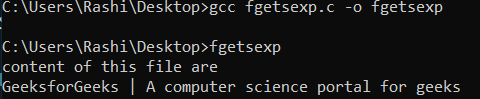fscanf()

fscanf() reads formatted input from a stream.

Syntax:

int fscanf(FILE *ptr, const char *format, …)

Approach:

• fscanf reads formatted data from the files and stores it in variables.
• The data in the buffer is printed on the console till the end of the file is reached.

## C++

 `// C program to implement``// the above approach``#include ` `// Driver code``int` `main()``{``    ``FILE``* ptr = ``fopen``(``"abc.txt"``, ``"r"``);``    ``if` `(ptr == NULL) {``        ``printf``(``"no such file."``);``        ``return` `0;``    ``}` `    ``/* Assuming that test.txt has content``       ``in below format``    ``NAME AGE CITY``    ``abc     12 hyderbad``    ``bef     25 delhi``    ``cce     65 bangalore */``    ``char` `buf;``    ``while` `(``fscanf``(ptr, ``"%*s %*s %s "``,``                  ``buf)``           ``== 1)``        ``printf``(``"%s\n"``, buf);` `    ``return` `0;``}`

Output: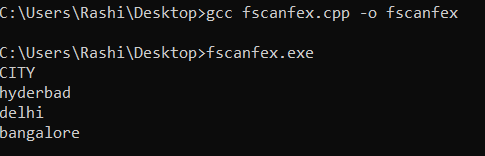fread() makes it easier to read blocks of data from a file. For instance, in the case of reading a structure from the file, it becomes an easy job to read using fread.

Syntax:

size_t fread(void *ptr, size_t size, size_t nmemb, FILE *stream)

ptr: This is the pointer to a block of memory with a minimum size of size*nmemb bytes.
size: This is the size in bytes of each element to be read.
nmemb: This is the number of elements, each one with a size of size bytes.
stream: This is the pointer to a FILE object that specifies an input stream.

Approach:

• It first, reads the count number of objects, each one with a size of size bytes from the given input stream.
• The total amount of bytes reads if successful is (size*count).
• According to the no. of characters read, the indicator file position is incremented.
• If the objects read are not trivially copy-able, then the behavior is undefined and if the value of size or count is equal to zero, then this program will simply return 0.

## C++

 `// C program to implement``// the above approach``#include ``#include ``#include ` `// Structure to store``// course details``struct` `Course {``    ``char` `cname;``    ``char` `sdate;``};` `// Driver code``int` `main()``{``    ``FILE``* of;``    ``of = ``fopen``(``"test.txt"``, ``"w"``);``    ``if` `(of == NULL) {``        ``fprintf``(stderr,``                ``"\nError to open the file\n"``);``        ``exit``(1);``    ``}` `    ``struct` `Course inp1 = { ``"Algorithms"``,``                           ``"30OCT"` `};``    ``struct` `Course inp2 = { ``"DataStructures"``,``                           ``"28SEPT"` `};``    ``struct` `Course inp3 = { ``"Programming"``,``                           ``"1NOV"` `};``    ``fwrite``(&inp1, ``sizeof``(``struct` `Course),``           ``1, of);``    ``fwrite``(&inp2, ``sizeof``(``struct` `Course),``           ``1, of);``    ``fwrite``(&inp3, ``sizeof``(``struct` `Course),``           ``1, of);``    ``if` `(``fwrite` `!= 0)``        ``printf``(``"Contents to file written successfully !\n"``);``    ``else``        ``printf``(``"Error writing file !\n"``);``    ``fclose``(of);` `    ``// File pointer to read from file``    ``FILE``* inf;``    ``struct` `Course inp;``    ``inf = ``fopen``(``"test.txt"``, ``"r"``);` `    ``if` `(inf == NULL) {``        ``fprintf``(stderr,``                ``"\nError to open the file\n"``);``        ``exit``(1);``    ``}` `    ``while` `(``fread``(&inp, ``sizeof``(``struct` `Course),``                 ``1, inf))``        ``printf``(``"Course Name = %s Started = %s\n"``,``               ``inp.cname, inp.sdate);``    ``fclose``(inf);``}`

Output: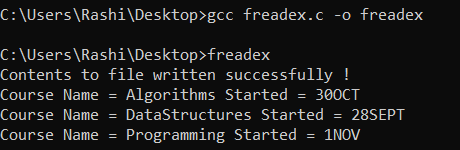My Personal Notes arrow_drop_up